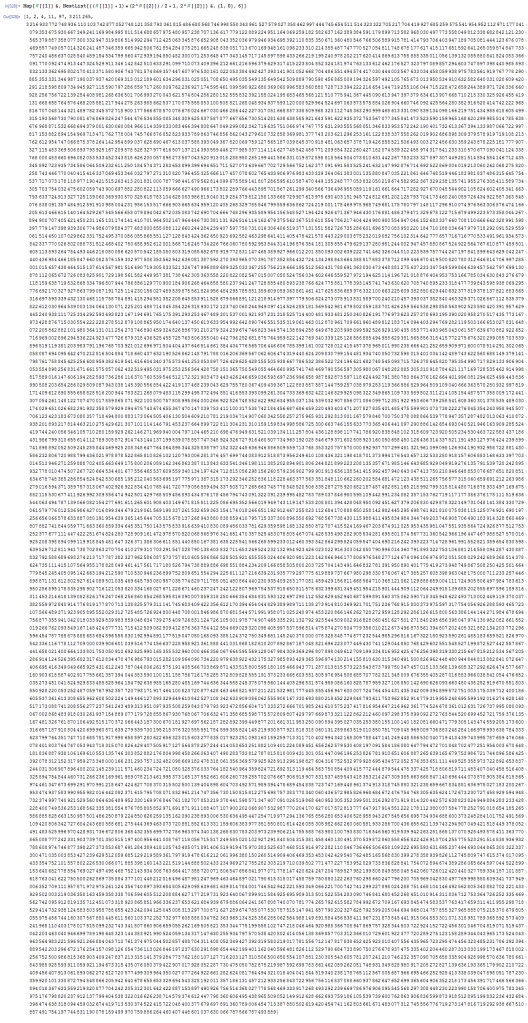# Growth of recursive string substitution

A Lindenmeyer system, or L-system, involves a recursive procedure applied to a string of symbols, where each symbol in the string is simultaneously replaced with a string, dependent on that symbol. For example, one of my favourite examples involves Easter eggs and rabbits, with the following rewrite rules:

"E" --> "R" (Easter egg hatches into a rabbit.)
"R" --> "ER" (Rabbit lays an Easter egg.)

Beginning with a single egg, the following growth pattern is observed:

"E" --> "R" --> "ER" --> "RER" --> "ERRER" --> ...

It’s trivial to show that the number of symbols in a particular iteration is given by the Fibonacci sequence. Indeed, for any L-system, we can obtain a recurrence relation for the number of characters, which can then be turned into a closed form involving powers of a particular ‘transition matrix’, which can be converted into an even more closed form by diagonalising the matrix. In this case, the closed form is Binet’s formula:$\dfrac{\phi^n - \psi^n}{\phi - \psi}$

where φ and ψ are the roots of the equation x² − x − 1 = 0.

### Audioactive decay

The lengths of strings produced by an L-system follow a basic linear recurrence relation, so they’re quite easy to calculate. A less obvious growth is that undertaken by Conway’s audioactive decay sequence. This begins with a single ‘1’, which is repeatedly run-length encoded:

• 1
• 11
• 21
• 1211
• 111221
• 312211

For example, the third string 111221 contains 3 ‘1’s, followed by 2 ‘2’s and 1 ‘1’, so the next term is 312211. Since adjacent digits can interact, the behaviour is less predictable than that of an L-system. It transpires, however, that after 24 iterations any string will ‘decay’ into a disjoint union of non-interacting ‘elements’, of which there are 92. Treating these elements as individual ‘symbols’, the audioactive decay rule is just a complicated L-system! The dominant eigenvalue is Conway’s constant, the positive real root of a particular degree-71 polynomial:Doron Zeilberger and his pet computer Shalosh B. Ekhad proved this in their paper on the subject. He mentions how it’s a posteriori trivial, since it takes no effort to write a computer program to verify that strings eventually decay into a meta-L-system, but not a priori trivial — similar rulesets (certain Post-Tag systems) can have no computable closed form, in which case the computer program would run forever and fail to find a proof.

### Double-exponential growth and bangbangs

If a character can be replaced with the previous string, we can have double-exponential growth. For instance, the following rules:

X --> $O --> O (where$ indicates the entirety of the previous string) applied to the initial string XOX gives the following sequence:

XOX --> XOXOXOX --> XOXOXOXOXOXOXOXOXOXOXOXOXOXOXOX --> ...

where each term has 2^2^n ‘X’s.

Of course, this is not a particularly interesting example, since it just creates alternating strings of one-dimensional noughts and crosses. A far more exciting sequence, sent to me by Volker Grabsch, arises when one experiments with the recursive functionality of Unix/Linux, which has a ‘repeat previous instruction’ command, known as a bangbang and represented by two adjacent exclamation marks.

Shadab Ahmed discovered that the resulting behaviour is quite complicated, since single quotes and double quotes can interchange roles as being string delimiters and string contents, and they have a non-trivial effect on which bangbangs are expressed at any time. Volker Grabsch wrote an algorithm to emulate this process, calculating further terms in the sequence and creating an OEIS entry for the number of bangbangs in the nth iteration. Robin Houston then ingeniously used polynomials with exponents in the symmetric group to contain the information in the strings, yielding a simple recurrence relation and an efficient way to compute further terms.

What’s remarkable is that all of this collaborative research, from initial contemplation of the problem to final recurrence relation, took place just two days ago.

### Tetrational growth

In a previous article, I mentioned the finite stages of the von Neumann universe, and wondered how many pairs of braces appear in each stage. It is trivial to see that it each term is an asymptotically exponential function of the previous term, and not too difficult (by considering how many braces an average subset contains) to derive a straightforward recurrence relation.Interestingly, this was already on the OEIS under a different definition (based on rooted trees). I decided to add the next term to the b-file, even though they don’t generally like 20000-digit entries…

This entry was posted in Uncategorized. Bookmark the permalink.

### 0 Responses to Growth of recursive string substitution

1.wojowu says:

In “Tetrational growth” section you wrote ” It is trivial to see that it is doubly-exponential…”, and probably meant exponential there. By the way, what is identity tree you mentioned in OEIS entry?

2.apgoucher says:

Oops, I meant `tetrational’ (as implied by the subtitle), and replaced it with an explanation. It is much faster than either exponential or doubly-exponential.

•wojowu says:

I meant tetrational in my message too 😛 I wonder if there is any “natural” function which grows at pentational rate.

3.talithin says:

You might like to know that the first L-system that you mentioned is more commonly known as the ‘Fibonacci word’ (http://en.wikipedia.org/wiki/Fibonacci_word) and it can be generated in many different ways. In particular, it is a Sturmian word (http://en.wikipedia.org/wiki/Sturmian_word), a class of words on two symbols which is particularly well studied.

4.Volker Grabsch says:

It is funny that you are also mentioning the audioactive decay, because I just proposed any algorithm that tries to compute this without string replacement operations:

Is is not yet approved by OEIS, so here’s the current draft:

https://oeis.org/history/view?seq=A005150&v=82

•Volker Grabsch says:

For clarification: Of course the sequence is already accepted by OEIS. It’s just the latest addition of my new implementation that’s not yet accepted.